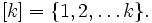# Boolean-valued function

(diff) ← Older revision | Latest revision (diff) | Newer revision → (diff)
Jump to: navigation, search

This page belongs to resource collections on Logic and Inquiry.

A boolean-valued function is a function of the typewhereis an arbitrary set and where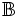is a boolean domain.

In the formal sciencesmathematics, mathematical logic, statistics — and their applied disciplines, a boolean-valued function may also be referred to as a characteristic function, indicator function, predicate, or proposition. In all of these uses it is understood that the various terms refer to a mathematical object and not the corresponding semiotic sign or syntactic expression.

In formal semantic theories of truth, a truth predicate is a predicate on the sentences of a formal language, interpreted for logic, that formalizes the intuitive concept that is normally expressed by saying that a sentence is true. A truth predicate may have additional domains beyond the formal language domain, if that is what is required to determine a final truth value.

## Examples

A binary sequence is a boolean-valued function, where. In other words,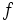is an infinite sequence of 0's and 1's.

A binary sequence of length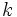is a boolean-valued function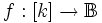, where